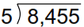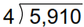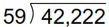# Grade 6 Multiplication & Division Worksheets

## Multiplication and division math worksheets

These grade 6 math worksheets give additional computational practice, particularly in column form multiplication and long division.

## Multiplication

Multiply in parts (distributive property) 5 x 104 =
Multiply 5-digit by 1-digit 36,276
x 3

Multiply 3-digit by 2-digit 164
x 22

Multiply 4-digit by 2-digit 1,164
x 22

Multiply 3-digit by 3-digit 164
x 322

Multiply 4-digit by 3-digit 1,164
x 322

## Long Division

Division by 1-digit, no remainderDivision by 1-digit, with remainderLong division by 2-digit divisorsMissing dividend or divisor problems 2,772 ÷ _____ = 66
Missing factor problems (solve by long division) 1,520 = _____ x 19